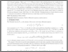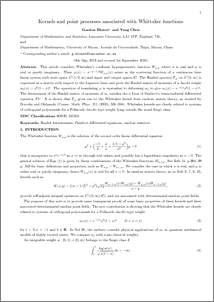# Kernels and point processes associated with Whittaker functions

Blower, Gordon and Chen, Yang (2016) Kernels and point processes associated with Whittaker functions. Journal of Mathematical Physics, 57 (9). ISSN 0022-2488Preview
PDF (JMPWhittakerrevised)
JMPWhittakerrevised.pdf - Accepted Version

## Abstract

This article considers Whittaker's confluent hypergeometric function $W_{\kappa ,\mu }$ where $\kappa$ is real and $\mu$ is real or purely imaginary. Then $\varphi (x)=x^{-\mu -1/2}W_{\kappa ,\mu }(x)$ arises as the scattering function of a continuous time linear system with state space $L^2(1/2, \infty )$ and input and output spaces ${\bf C}$. The Hankel operator $\Gamma_\varphi$ on $L^2(0, \infty )$ is expressed as a matrix with respect to the Laguerre basis and gives the Hankel matrix of moments of a Jacobi weight $w_0(x)=x^b(1-x)^a$. The operation of translating $\varphi$ is equivalent to deforming $w_0$ to give $w_t (x)=e^{-t/x}x^b(1-x)^a$. The determinant of the Hankel matrix of moments of $w_\varepsilon$ satisfies the $\sigma$ form of Painlev\'e's transcendental differential equation $PV$. It is shown that $\Gamma_\varphi$ gives rise to the Whittaker kernel from random matrix theory, as studied by Borodin and Olshanski (Comm. Math. Phys. 211 (2000), 335--358). Whittaker kernels are closely related to systems of orthogonal polynomials for a Pollaczek--Jacobi type weight lying outside the usual Szeg\"o class.\par

Item Type:
Journal Article
Journal or Publication Title:
Journal of Mathematical Physics
Copyright 2016 American Institute of Physics. The following article appeared in Journal of Mathematical Physics, 57, 2016 and may be found at http://dx.doi.org/10.1063/1.4963170 This article may be downloaded for personal use only. Any other use requires prior permission of the author and the American Institute of Physics. This is a substantially revised version of paper with same authors and title which was previously place on AvXiv and Lancaster University Pure repositories.
Uncontrolled Keywords:
/dk/atira/pure/subjectarea/asjc/3100/3109
Subjects:
Departments:
ID Code:
81561
Deposited By:
Deposited On:
14 Sep 2016 08:12
Refereed?:
Yes
Published?:
Published